# How to denote cross product(a ⨯ b) in LaTeX?

You all know how to represent cross products in writing. You need to use multiplication symbols to write cross products in vectors. For which you need to use \times command in latex.

Suppose here a and b are two vectors, then their cross product will be

\documentclass{article}
\begin{document}
$$\vec{a}\times\vec{b}$$
\end{document}


Output :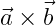Two commands are used to represent this vector cross product in latex. The first is \vec{} in which if a single character is passed as an argument it returns an arrow mark on that single character. And the second is \times in which there is no need to pass any argument, which will return you the multiplication symbol.

The result of the cross product always represents the vector. And this result is written in the form of unit vector and matrix.

## Cross product in the form of unit vector

In this case the cross product is expressed in unit vectors with magnitude.

To represent a character by mod, you have to use the \left| and \right| commands and pass the character through both.

\documentclass{article}
\begin{document}
$$\vec{a}\times\vec{b} =\left| \vec{a}\times\vec{b} \right |\hat{n}$$
\end{document}


Output :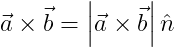Also when the unit vector is written you need to use the \hat{} command which will return a cap on a character.

Second, you can write the value of the cross product in the form of sinθ. For example

Here for sinθ, you need to use the two latex commands \sin and \theta. And \theta command will return you theta symbol.

\documentclass{article}
\begin{document}
$$\vec{a}\times\vec{b} =|\vec{a}||\vec{b}|\sin\theta\hat{n}$$
\end{document}


Output :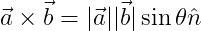## Cross Product in the form of Matrix

Before writing a cross product in the form of a matrix, you need to know how the matrix is ​​defined in latex. There are different types of matrix, but in this tutorial, we will discuss one type of matrix whose elements will be enclosed in parentheses.

Basic syntax of a matrix without any type of bracket

$\begin{matrix} .... .... \end{matrix}$

And since I will use the parenthesis matrix here, you have to type pmatrix instead of matrix in the \begin{} and \end{} commands.

$\begin{pmatrix} .... .... \end{pmatrix}$

You are creating the basic syntax of the matrix. Again you have to give the element in the matrix. For this, you need to use two symbols & and \\. The & symbol will create a gap between two elements and & symbol will create a new line.

\documentclass{article}
\usepackage{amsmath}
\begin{document}
$$\begin{pmatrix} a & b \\ c & d \end{pmatrix}$$
\end{document}

Output :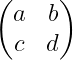You notice the output above where the & symbol has created spaces between (a, b) and (c, d) and the \\ symbol has created new lines between the arranged elements in a row.

Hopefully, this time if I write the cross product of two-position vectors in the form of a matrix then you will not have any problem in understanding. So,

\documentclass{article}
\usepackage{amsmath}
\begin{document}
$$\vec{a}\times\vec{b}=\begin{pmatrix} \hat{i} & \hat{j} & \hat{k}\\ x_{1} & y_{1} & z_{1} \\ x_{2} & y_{2} & z_{2} \end{pmatrix}$$
\end{document}

Output :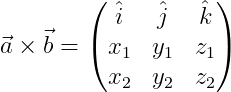1. How do you write a unit vector in the form of a cross vector?

\documentclass{article}
\begin{document}
$$\hat{n}=\frac{\vec{a}\times\vec{b}}{\left | \vec{a}\times\vec{b}\right |}$$
\end{document}

Output :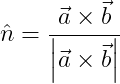Notice the latex code above. The \frac{}{} command is used for fractions and you always have to pass two arguments for numerator and denominator in this command.

2. How do you define a triple product with latex?

Triple products are always written with a combination of vector dot and cross products.

\documentclass{article}
\begin{document}
$$\vec{a}\cdot \left(\vec{b}\times\vec{c}\right )$$
\end{document}


Output :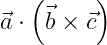You need to use the \cdot command for the dot symbol. And for parenthesis, you need to pass arguments between the \left ( and \right ) commands.

## Conclusion

This tutorial discusses latex cross products in depth. You could define these latex equations by calling other external packages. But here the genuine method is shared with you.

And since Latex has a variety of commands, don’t be confused. There are many commands in which you do not have to pass any argument and there are many commands in which you have to pass more than one argument. Thanks#### Md Jidan Mondal

LaTeX expert with over 10 years of experience in document preparation and typesetting. Specializes in creating professional documents, reports, and presentations using LaTeX.